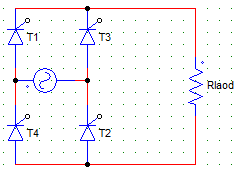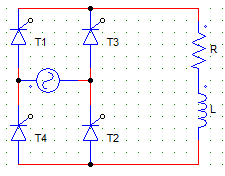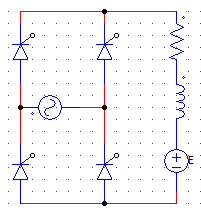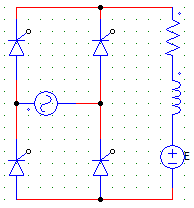Test: Single Phase FW AC-DC - 2

# Test: Single Phase FW AC-DC - 2

Test Description

## 20 Questions MCQ Test Power Electronics | Test: Single Phase FW AC-DC - 2

Test: Single Phase FW AC-DC - 2 for Electrical Engineering (EE) 2023 is part of Power Electronics preparation. The Test: Single Phase FW AC-DC - 2 questions and answers have been prepared according to the Electrical Engineering (EE) exam syllabus.The Test: Single Phase FW AC-DC - 2 MCQs are made for Electrical Engineering (EE) 2023 Exam. Find important definitions, questions, notes, meanings, examples, exercises, MCQs and online tests for Test: Single Phase FW AC-DC - 2 below.
Solutions of Test: Single Phase FW AC-DC - 2 questions in English are available as part of our Power Electronics for Electrical Engineering (EE) & Test: Single Phase FW AC-DC - 2 solutions in Hindi for Power Electronics course. Download more important topics, notes, lectures and mock test series for Electrical Engineering (EE) Exam by signing up for free. Attempt Test: Single Phase FW AC-DC - 2 | 20 questions in 20 minutes | Mock test for Electrical Engineering (EE) preparation | Free important questions MCQ to study Power Electronics for Electrical Engineering (EE) Exam | Download free PDF with solutions
 1 Crore+ students have signed up on EduRev. Have you?
Test: Single Phase FW AC-DC - 2 - Question 1

### An SCR has the peak forward voltage = 1000 V. Find the maximum voltage that the SCR can handle if employed in a B-2 type full controlled converter circuit. Use factor of safety (FOS) = 2.5

Detailed Solution for Test: Single Phase FW AC-DC - 2 - Question 1

In M-2 type configuration maximum voltage handled is Vm
Therefore, 1000/2.5 = 400 V.

Test: Single Phase FW AC-DC - 2 - Question 2

### A single-phase full converter bridge, is connected to a RLE load. The source has a rms voltage of 230 V and the average load current is 10 A. Find the firing angle for which the power flows from AC source to the DC load. Consider E = 120 V, R = 0.4 Ω.

Detailed Solution for Test: Single Phase FW AC-DC - 2 - Question 2

We have,
Vo = E + IR
Vo = 2Vm/π x cosα
Substitute the given values to discover α = 53.208°.

Test: Single Phase FW AC-DC - 2 - Question 3

### A single-phase full converter bridge, is connected to a RLE load. The source has a rms voltage of 230 V and the average load current is 10 A. Find the firing angle for which the power flows from the DC load to the AC source. Consider E = 120 V, R = 0.4 Ω, L = 2 Henry.

Detailed Solution for Test: Single Phase FW AC-DC - 2 - Question 3

We have,
Vo = E + IR
Vo = 2Vm/π x cosα . . . (i)
Substitute the given values to discover α = 124°
Note that as the power is flowing from DC to AC E has to be negative.
E = -120V.
Use 1 to find the firing angle.

Test: Single Phase FW AC-DC - 2 - Question 4

For the below shown converter configuration, find the expression for the average value of voltage across the resister R with Vs = Vm sinωt and firing angle = α.Detailed Solution for Test: Single Phase FW AC-DC - 2 - Question 4

Vo = Vo = 1/π x [∫ Vm sinωt d(ωt)] where the integration would run from α to π.

Test: Single Phase FW AC-DC - 2 - Question 5

A single phase full controlled bridge converter is connected to load having R = 0.4 Ω, E = 120, L = 0.2 mH, Vs = 230V. The RMS value of load current is 10 A for a firing angle of 53.21°. Find the input power factor.

Detailed Solution for Test: Single Phase FW AC-DC - 2 - Question 5

Io = Irms = 10A
Vs x Irms x cosɸ = E Io + Irms2 R.

Test: Single Phase FW AC-DC - 2 - Question 6

A single phase full converter feeds power to a RLE load with R = 6 Ω and E = 60 V. Find the average value of the load current when the supply voltage is 230 V rms AC and a firing angle = 50°.

Detailed Solution for Test: Single Phase FW AC-DC - 2 - Question 6

Vo = 2Vm/π cosα = 133.084 V
I = Vo – E/R = 133.084-60 / 6 = 12.181 A.

Test: Single Phase FW AC-DC - 2 - Question 7

A single phase full wave mid-point SCR converter, uses a 230/200 V transformer with the centre tap on the secondary side. The P.I.V. per SCR is

Detailed Solution for Test: Single Phase FW AC-DC - 2 - Question 7

PIV for M-2 is Vm = √2Vs = √2 x 200.

Test: Single Phase FW AC-DC - 2 - Question 8

In a single phase full converter, for discontinuous load current and extinction angle β > π, each SCR conducts for

Detailed Solution for Test: Single Phase FW AC-DC - 2 - Question 8

Each device would conduct for β-α.

Test: Single Phase FW AC-DC - 2 - Question 9

A single-phase two pulse converter feeds RL load with a sufficient smoothing such that the load current does not fall to zero. If the resistance of the load circuit is increased then the

Detailed Solution for Test: Single Phase FW AC-DC - 2 - Question 9

If the resistance of the load circuit is increased then the ripple content of current increases.

Test: Single Phase FW AC-DC - 2 - Question 10

In case of controlled rectifiers, the nature of the load current (continues or discontinuous) depends upon the

Detailed Solution for Test: Single Phase FW AC-DC - 2 - Question 10

It depends on both as firing angle will decide how fast and how much current flows. The load R, Rl or RLE can also effect the current depending upon the values of L and E.

Test: Single Phase FW AC-DC - 2 - Question 11

In the circuit shown below, find the expression of average output voltage if due to some faults the SCR T3 gets permanently open circuited.Detailed Solution for Test: Single Phase FW AC-DC - 2 - Question 11

If one of the device gets O.C the circuit would behave just like a half-wave rectifier. Only Two SCRs T1 & T2 will operate, even T4 is useless in this case.
Vo = 1/2π x [ ∫ Vm sinωt d(ωt) ] where the integration would run from α to π+α.

Test: Single Phase FW AC-DC - 2 - Question 12

A single phase full converter, feeds power to a RLE load with R = 6 Ω and E = 60 V. The supply voltage is 230 V AC and the firing angle is 50°. Find the power delivered to the battery.

Detailed Solution for Test: Single Phase FW AC-DC - 2 - Question 12

Vo = 2Vm/π cosα = 133.084 V
I = Vo – E/R = 133.084-60 / 6 = 12.181 A
Power delivered = E x I = 60 x 12.181 = 730.86 Watts.

Test: Single Phase FW AC-DC - 2 - Question 13

In a single phase full converter with resistive load and firing angle α, the load current is

Detailed Solution for Test: Single Phase FW AC-DC - 2 - Question 13

The current starts to flow from α, π+α, …

Test: Single Phase FW AC-DC - 2 - Question 14

In a single phase semi converter with resistive load and a firing angle α, each SCR and freewheeling diode would conduct for

Detailed Solution for Test: Single Phase FW AC-DC - 2 - Question 14

FD does not come into the picture for resistive loads.

Test: Single Phase FW AC-DC - 2 - Question 15

A single phase full converter has average & peak voltage values of 133 V and 325 V respectively. Find the value of the firing angle.

Detailed Solution for Test: Single Phase FW AC-DC - 2 - Question 15

Vm = 325V
Vo = 2Vm/π cosα = 133 V.

Test: Single Phase FW AC-DC - 2 - Question 16

A freewheeling diode placed across a RL load provides

Detailed Solution for Test: Single Phase FW AC-DC - 2 - Question 16

FD improves efficiency by providing freewheeling action which increase the power delivered to the load

Test: Single Phase FW AC-DC - 2 - Question 17

A single phase full converter has discontinues load current. The converter is supplying a dc shunt motor (R-L-E) load. When the current falls to zero the output voltage is

Detailed Solution for Test: Single Phase FW AC-DC - 2 - Question 17

As the load is RLE output voltage will always exists even if the current goes to zero due to E.

Test: Single Phase FW AC-DC - 2 - Question 18

A single phase full converter has discontinues load current. The converter is supplying a DC shunt motor (R-L-E) load. When the current falls to zeroDetailed Solution for Test: Single Phase FW AC-DC - 2 - Question 18

The current falls to zero when the L has discharged completely and the devices are not yet fired.

Test: Single Phase FW AC-DC - 2 - Question 19

For the circuit shown below to act as a line commutated inverterDetailed Solution for Test: Single Phase FW AC-DC - 2 - Question 19

For inverter operation the firing angle should be above 180 and E should be reversed.

Test: Single Phase FW AC-DC - 2 - Question 20

If in the below given converter configuration, any 3 of the 4 SCRs are replaced by diodes

Detailed Solution for Test: Single Phase FW AC-DC - 2 - Question 20

If diodes are put in place of any 3 SCRs the conduction angle would increase and hence the voltage.

## Power Electronics

5 videos|39 docs|63 tests
 Use Code STAYHOME200 and get INR 200 additional OFF Use Coupon Code
Information about Test: Single Phase FW AC-DC - 2 Page
In this test you can find the Exam questions for Test: Single Phase FW AC-DC - 2 solved & explained in the simplest way possible. Besides giving Questions and answers for Test: Single Phase FW AC-DC - 2, EduRev gives you an ample number of Online tests for practice

## Power Electronics

5 videos|39 docs|63 tests